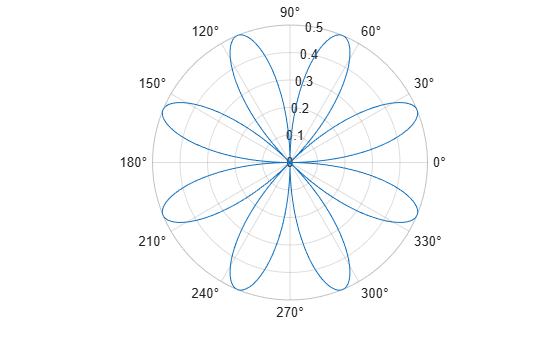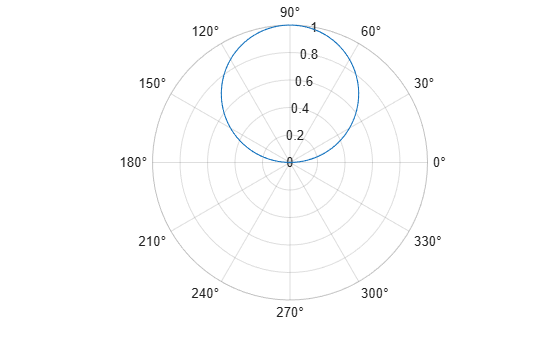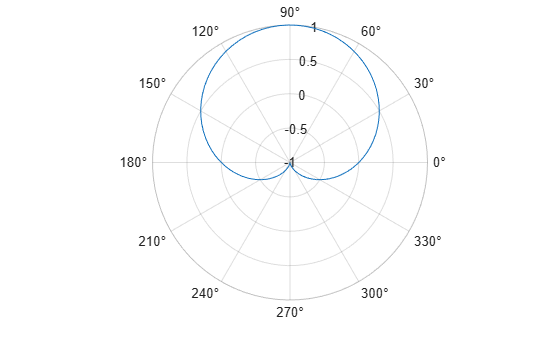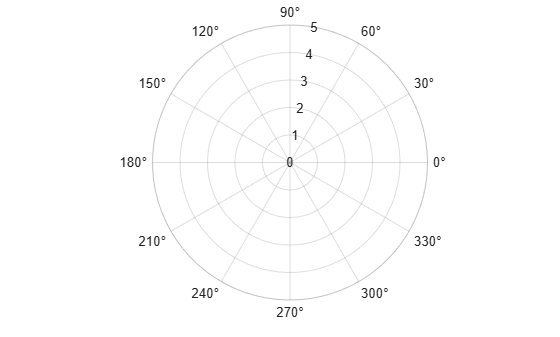# rlim

Set or query r-axis limits for polar axes

## Syntax

``rlim(limits)``
``rlim(limitmode)``
``rl = rlim``
``m = rlim("mode")``
``___ = rlim(pax,___)``

## Description

### Specify Limits

example

````rlim(limits)` specifies the r-axis limits for the current polar axes. Specify `limits` as a two-element vector of the form ```[rmin rmax]```, where `rmax` is a numeric value greater than `rmin`.```

example

````rlim(limitmode)` specifies automatic or manual limit selection. The `limitmode` can have either of two values: `"auto"` — Enable automatic limit selection. MATLAB® chooses the r-axis limits.`"manual"` — Freeze the r-axis limits at their current value. Use this option if you want to retain the current limits when adding new data to the polar axes using the `hold on` command. ```

### Query Limits

example

````rl = rlim` returns a two-element vector containing the limits for the current polar axes.```
````m = rlim("mode")` returns the current value of the limits mode, which is either `'auto'` or `'manual'`. By default, the mode is automatic unless you specify limits or set the mode to manual.```

### Specify Target Axes

example

````___ = rlim(pax,___)` uses the polar axes specified by `pax` instead of the current polar axes. Specify `pax` as the first input argument. Include additional input or output arguments only if the original syntax supported them.```

## Examples

collapse all

Create a polar plot and change the r-axis limits.

```theta = 0:0.01:2*pi; rho = sin(2*theta).*cos(2*theta); polarplot(theta,rho) rlim([0 1])```Set the limits back to the original values.

`rlim('auto')`Create a polar plot using negative radius values. By default, `polarplot` reflects negative values through the origin.

```theta = linspace(0,2*pi); rho = sin(theta); polarplot(theta,rho)```Change the limits of the r-axis so it ranges from -1 to 1.

`rlim([-1 1])`Create a polar plot and return the r-axis limits.

```theta = 0:0.01:2*pi; rho = sin(2*theta).*cos(2*theta); polarplot(theta,rho)````rl = rlim`
```rl = 1×2 0 0.5000 ```

Set the limits for a specific polar axes by specifying the polar axes object as the first input to `rlim`. Otherwise, `rlim` sets the limits for the current axes.

```pax = polaraxes; rlim(pax,[0 5])```## Input Arguments

collapse all

Minimum and maximum limits, specified as a two-element vector of the form `[rmin rmax]`, where `rmax` is a numeric value greater than `rmin`. You can specify both limits, or specify one limit and let MATLAB automatically calculate the other.

• To automatically set the minimum limit to the minimum data value, specify the first element as `-inf`, for example, `rlim([-inf 0])`.

• To automatically set the maximum limit to the maximum data value, specify the second element as `inf`, for example, `rlim([0 inf])`.

When you specify the limits, the `RLim` property for the polar axes object updates to the specified values and the `RLimMode` property changes to `'manual'`.

Example: `rlim([0 1])`

Limit mode, specified as one of the following values:

• `"auto"` — Enable automatic limit selection, which is based on the total span of the data. If you plot into the axes multiple times, the limits update to encompass all the data. You can use this option if you change the limits and want to set them back to the default values.

• `"manual"` — Freeze the limits at the current values. Use this option if you want to retain the current limits when adding new data to the axes using the ```hold on``` command.

When you specify this argument, MATLAB sets the `RLimMode` property of the axes to the value you specify. However, the `RLimMode` property changes to `"manual"` whenever you set the r-axis limits explicitly, either by calling `rlim(limits)`, or by setting the value of the `RLim` property on the axes.

Polar axes, or an array of polar axes. If you do not specify this argument, then `rlim` sets the limits for the current axes (provided that the current axes is a polar axes object).

## Output Arguments

collapse all

Current limits, returned as a two-element vector of the form `[rmin rmax]`. Querying the limits returns the value of the `RLim` property for the polar axes object.

Current limits mode, returned as one of these values:

• `'auto'` — The limits automatically update to reflect changes in the data.

• `'manual'` — The limits do not automatically update to reflect changes in the data.

Querying the r-axis limits mode returns the value of the `RLimMode` property for the polar axes object.

## Version History

Introduced in R2016a